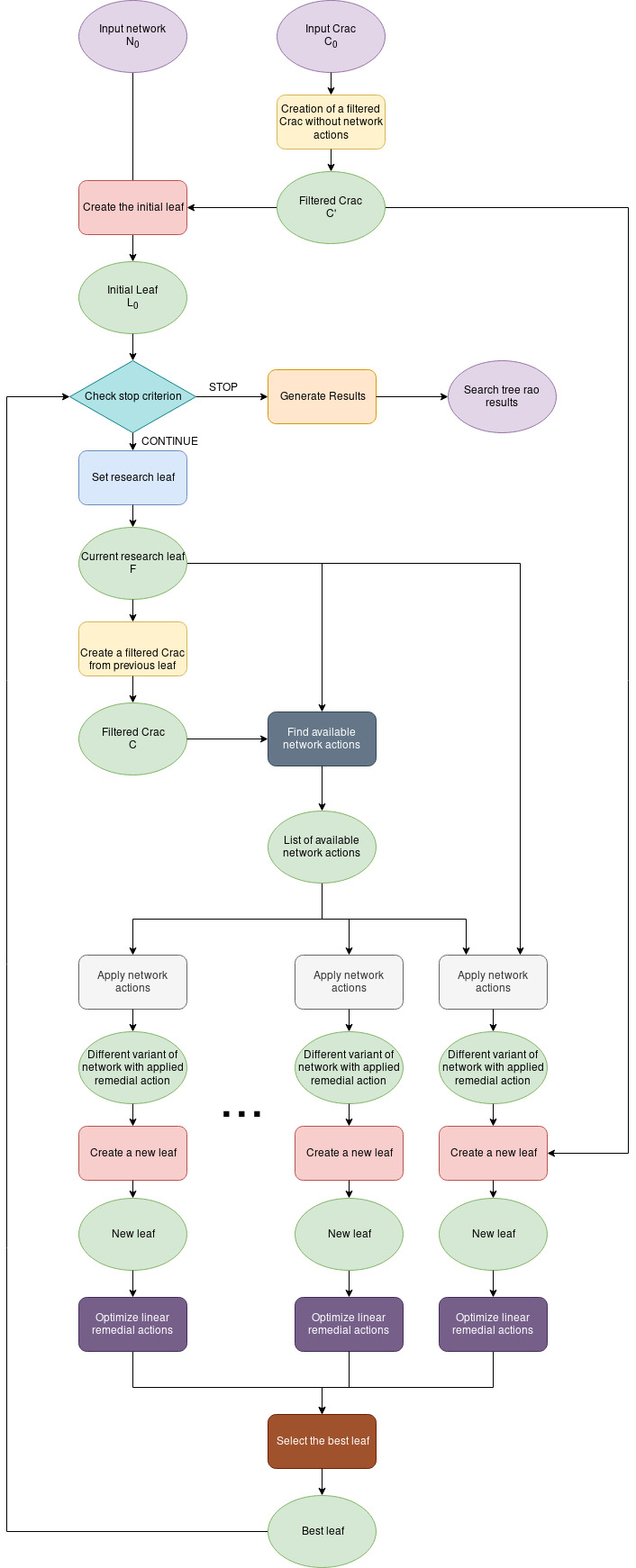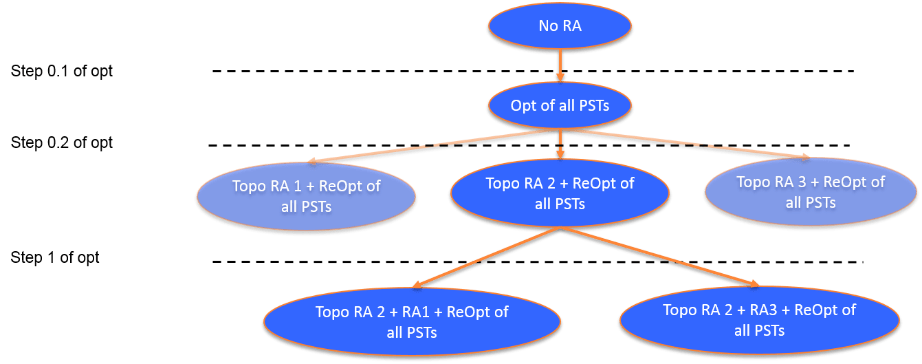# CASTOR

Contents

CASTOR (CAlculation with Scalable and Transparent OptimiseR) is initially designed to optimise non-costly remedial actions (including tripping of generation units where applicable). It relies on a search-tree algorithm which is explained in this page.

Unlike purely linear optimisation, a search-tree algorithm does not neglect the non-linearity of efficient remedial actions such as curative and preventive topological actions. Incorrect modeling of remedial actions such as topological remedial actions could lead to over or underestimate their impact and then result in a biased network situation that could endanger the system security.

So far, the search-tree algorithm has proved for many years its relevance and efficiency on current daily operational processes on CWE, Northern Italy and SWE Capacity calculation.

For each topological remedial action applied, the search-tree will systematically optimise PST taps & HVDC setpoints by applying a linear optimisation. By considering both topological remedial actions and linear remedial actions at every step, instead of considering only one after the other, CASTOR’s results are optimal.

The optimisation problem is a non-convex and non-linear one, dealing with topology changes on the network, which represent discrete actions by definition. The problem treated by the optimiser is a combinatorial problem. Search-tree algorithms are commonly used for high complexity mathematical problems, containing combinatorial and discrete aspects.

Some remedial actions such as PSTs & HVDCs are treated via a linear optimisation. It should be noted that approximation of discrete PST taps treated as linear variables can be valid in DC; but CASTOR performs a security analysis after optimising them in order to ensure the security of the grid despite the approximation.

### Higher grid security

A search-tree algorithm will optimise all couples of critical branches and critical outages to define a set of remedial actions covering all these network states. This limits the impact of net position forecasts, which are highly uncertain because of the uncertainty in the power generation forecasts (renewables, unforeseeable events…).

For example, let’s assume there that is no available preventive remedial action (for simplicity) and focus on the CNEC with the minimum relative margin (let’s call it CNE1C1).
The optimiser will search for a curative remedial action increasing margin of CNE1C1. However, considering only CNE1C1 could be relevant if, and only if, the net position forecast is highly reliable and accurate. As stated before, this could not be acceptable for the security of the system. That is why the search tree will also identify optimised remedial actions for CNE1C2, CNE1C3…

### Remedial actions considered

For Capacity calculation processes, CASTOR considers the following remedial actions:

• Preventive Remedial Actions (fully shared) hereafter “PRA”
• Automatic Remedial Actions (shared after a critical outage) hereafter “ARA”. Note that these remedial actions are simulatedrather than optimised.
• Curative Remedial Actions (shared after a critical outage) hereafter “CRA”

### Linear Remedial actions

The impact of some types of remedial actions on flows could be considered to be linear: optimisation of HVDC setpoints and optimisation of generation unit setpoints. In addition to these, CASTOR also considers phase shifter transformers as linear remedial actions.1

A phase shifter transformer (PST) is defined by its range of acceptable tap settings. This acceptable tap setting can be defined by three different ways:

• a range of tap positions relatively to the neutral tap position of the PST (e-g +6/-6 around the neutral tap position)
• a range of tap positions relatively to the tap position of the PST as described in the grid model
• in curative: a range of tap positions relatively to the optimised tap position of the PST in preventive (e-g +4/-4 around the tap position selected in preventive)

### Non-linear remedial action

Topological and other discrete remedial actions are considered without any approximation, and can be optimised in both instants (preventive or curative). This is a non-exhaustive list of discrete remedial actions considered by CASTOR:

• Change of circuit breaker position (open/close): line opening or busbar coupler opening/closing
• Change of switch position

As a matter of clarification, connecting/disconnecting a generation unit can also be considered by CASTOR for Capacity calculation (without elements of costs).

### Objective function

#### For Flow-based Capacity calculation – minimum margin

The objective function is used to determine at each step of the search tree which remedial action is the best. A variant of it is also used when solving the linear optimisation problem.

The active flow $$F_i$$ on a CNEC $$i$$ is:

$\begin{equation} F_i = F_{ref_{0,i}} + ( \sum_{j \in J} \Delta\alpha_j * S_{j,i}) + \Delta F_i \end{equation}$

With:

• $$F_{ref_{0,i}}$$ the initial active transit on CNEC $$i$$
• $$J$$ the set of all linear remedial actions (LRA)
• $$S_{j,i}$$ the sensitivity of LRA $$j$$ on CNEC $$i$$

And:

• $$\Delta\alpha_j$$ variation of setpoint of LRA $$j$$ (between initial situation and final situation)
• $$\Delta F_i$$ impact of a set of applied non-linear RAs on CNEC $$i$$

Optimisation will try to maximize the objective function value, formulated as such:

$\begin{equation} OF = min_i(\rho_i * F_{max_i}-F_i) \end{equation}$

With :

• For the sake of readability, we consider in the rest of the explanation that the FRM value is already considered in F_{max_i}.
• and $$\rho_i = 1 \ \ \forall i\quad$$ if at least one CNEC is in overload
$$\rho_i = \frac{1}{\sum_{ma}|PTDF_{ma,i}|}\ \ \forall i\quad$$ if all margins are positive, with $$PTDF_{ma,i}$$ the PTDF of CNEC $$i$$ relatively to a commercial border (zone-to-zone) of the capacity calculation region = one commercial border in the capacity calculation region (e-g FR-BE)

The objective function of CASTOR is also configurable. The constraints taken into account within the optimisation are also configurable in order to comply with process specificities (i.e limitation of loop flows required for Flow based CORE Capacity Calculation).

#### For NTC Capacity calculation/CEP Validation

The objective function is :

$\begin{equation} OF = min_i(F_{max_i}-F_i) \end{equation}$

For the NTC Capacity calculation, only the positive margin stop criterion is activated. This is equivalent to an iterative security analysis at different values of commercial exchanges. It is also valid for the CEP Validation performed at national or regional level.

### Inputs

This algorithm acts on sets of states that share common remedial actions, also called perimeters.

The main inputs of the algorithm are:

• the network at the root of the perimeter,
• an extract of the original CRAC, containing only the remedial actions that are available in the given perimeter (filtered on usage rules).

### Stop criterion

For Flow based Capacity calculation, considering that the objective function is the minimum margin (meaning the minimum of all flow margins of every CNEC), there are currently two stop criteria, ie two ways to stop the search tree algorithm:

• when the minimum margin is positive, meaning that the network is secured (all the CNEC flows are under line thresholds): positive margin stop criterion. As mentioned above, for NTC Capacity calculation/ CEP Validation, this stop criterion is applied.
• when the the minimal margin on every CNEC cannot be increased anymore: maximum margin stop criterion.

These stop criteria only make sense for a minimum margin objective function (may it be absolute or relative).

### AlgorithmFor each iteration/step (a level of depth in tree):

• Determination of available remedial actions.
• Once the list of available remedial actions is defined, candidates are created. Each candidate corresponds to a grid situation, where one (or more) remedial actions are applied.
• A skippable optimisation of the linear remedial actions is done.
• A security analysis determines for each candidate the value of the objective function. The security analysis consists of a series of DC (or AC) load-flow computations (for each defined contingency).
• In order to maximise the objective function, values obtained for each candidate are compared: the candidate leading to the best increase of objective function value is selected. The remedial actions corresponding to this candidate are applied.

Note that if no candidate can increase the objective function value more than configurable value, the optimisation of the current studied perimeter (preventive/curative) stops. All candidates applied in previous steps/iterations of optimisation will be considered as final found remedial actions.

#### Usefulness of optimising linear remedial actions at every step

By default, CASTOR always studies the combination of PST and other linear remedial actions with each individual non-linear remedial action. This allows to better take into consideration the joint effect of non-linear remedial with available LRAs (in particular if the non-linear remedial action impacts significantly the sensitivity of available LRAs, typically if both actions are in close electrical vicinity).In this figure above, 3 non-linear remedial actions are available, as well as a list of PSTs (LRAs). Each non-linear remedial action is applied on the grid situation. However, prior to an assessment of the objective function value, sensitivities are assessed (with consideration of the applied non-linear action), and LRAs are optimised.

RA1 alone could have led to bigger objective function value than RA2 or RA3. However, when taking PSTs into account, RA2 with optimised PSTs is more efficient than other RA and optimised tap settings.

1. CASTOR offers different approximation levels for modelling PSTs. See the relevant RAO parameter for more information.